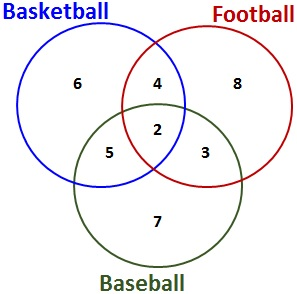© Ms. Garcia Math 7th Favorite Sports Survey Results GR30 answersAnswer the questions based on the Venn Diagram 1) How many students only like Basketball? 2) How many students only like Baseball? 6 7 Answer Answer 3) How many students only like Football? 4) How many students like both Football and Baseball? 8 3 Answer Answer 5) How many students like both Football and Basketball? 6) How many students like both Basketball and Baseball? 4 5 Answer Answer 7) How many students like Football, Basketball, and Baseball? 8) Find out the number of students who play sports 2 35 Answer Answer Name: ID: